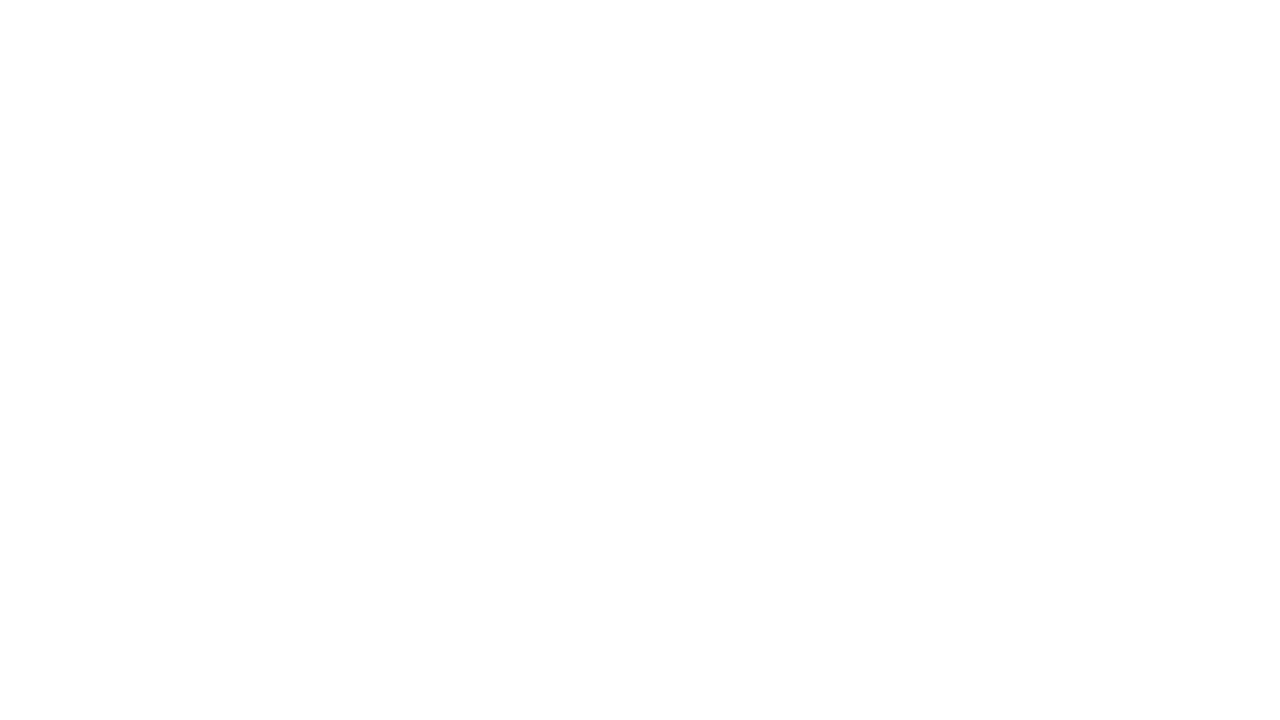in Artificial IntelligenceRegistration is open until December 20
* only for college students
Training course from the organizers
Curriculum
Lecture 01 - Introduction to Artificial Intelligence (AI)
- What is AI?
- The foundations of AI
- History of AI
- The State of the Art
- Strategies for solving problems

Lecture 02 - Solving problems by searching
- Examples of problems
- Searching for solutions
- Graph as a model for searching
- Uninformed search strategies

Lecture 03 - Heuristics
- Recap of searching and uninformed searching
- Informed (heuristic) search strategies

Lecture 04 - Local search algorithms and optimization problems
- Hill climbing
- Simulated Annealing

Lecture 05 - Genetic Algorithms
- Natural selection and evolution in computer science
- Representation of solutions
- Crossover and mutation
- STOPP criteria

Lecture 06 - Solving problems using logic
- Propositional logic
- First-order logic

Lecture 07 - Solving problems by using examples (learning)
- Forms of learning
- Supervised and unsupervised learning
- Decision trees

Lecture 08 - Regression and classification
Prerequisites
This course has been designed for students with the following skillset:

- Basic algorithmic thinking (pseudocode, use of loops, if-then-else structures, arrays, derived data structures);

- Basic logical thinking (boolean algebra, truth tables);

- Intermediate programming using any programming language (preferably but not mandatory Python and Java);

- Intermediate mathematics (basic probability, statistics, set operations and graphs).
After training
You will learn to apply complex algorithms for solving real-world problems.

In this course you also will learn what Artificial Intelligence (AI) is, explore use cases and applications of AI, understand AI concepts and terms like machine learning, deep learning and neural networks.

You will learn how to write a game bot. You will learn how to solve problems using the search method. You will learn to apply heuristic strategies and local search algorithms and genetic algorithms.
Contacts
+7(843) 203-92-53 (ext. 286)
ioai@innopolis.university
1, Universitetskaya Str., Innopolis, Russia